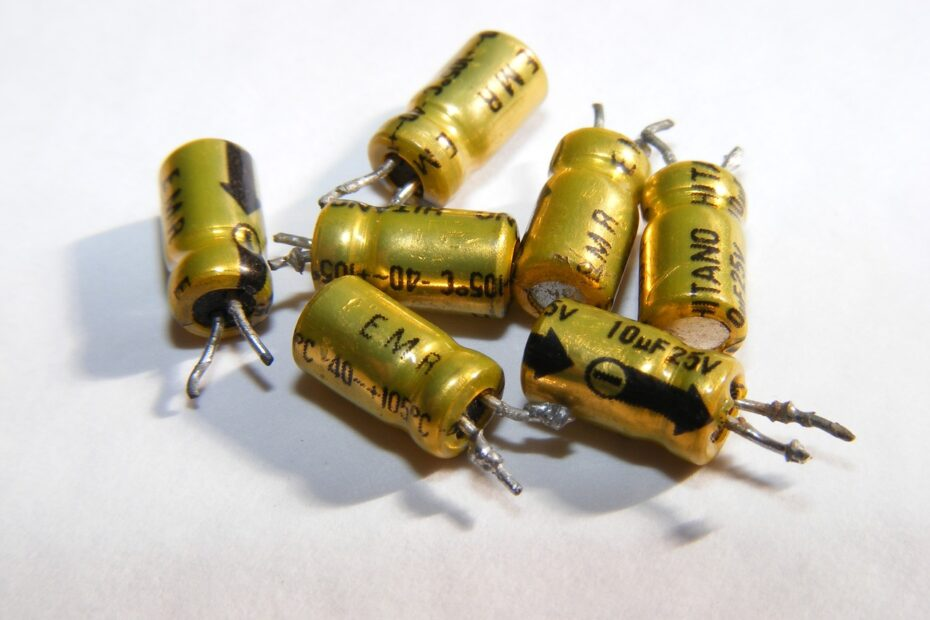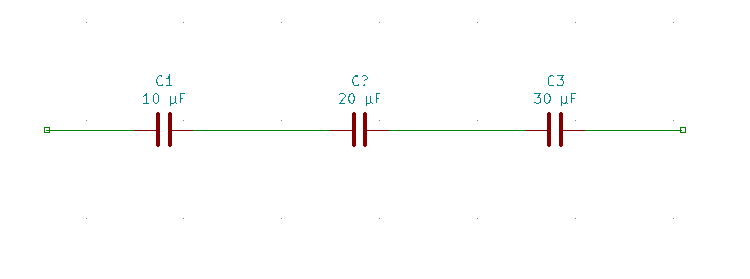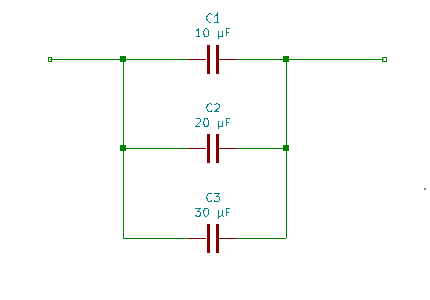# Calculating capacitors in series and parallel circuitsCapacitors are fundamental electrical components that store and release electrical energy. They find applications in various electronic devices, from simple circuits to complex systems. Understanding how to calculate capacitors in series and parallel circuits is crucial for designing and troubleshooting electronic circuits. In this tutorial, we will delve into the concepts and methods for calculating capacitors in series and parallel configurations.

## Basics of capacitance

Before we jump into series and parallel circuits, let’s review some fundamental concepts related to capacitors.

1.  Capacitance (C): Capacitance is the property of a capacitor to store electrical energy. It is measured in farads (F), with 1 farad equal to 1 coulomb of charge stored per volt of potential difference.

2.  Capacitor symbol: The standard symbol for a capacitor is two parallel lines separated by a gap.

3.  Charge and voltage: The charge (Q) on a capacitor is directly proportional to the voltage (V) across it, and the capacitance (C) of the capacitor: Q = C * V.

4.  Energy stored: The energy stored in a capacitor is given by the formula: U = 1/2 * C * V^2.

Now, let’s explore how capacitors behave in series and parallel configurations.

## Capacitors in series

### Connection

When capacitors are connected in series, they share the same current but have different voltages across them. They are connected end-to-end so that the positive terminal of one capacitor is connected to the negative terminal of the next.### Formula

To find the total capacitance of capacitors in series, you can use the reciprocal formula:

1 / C_total = 1 / C1 + 1 / C2 + 1 / C3 + …

Where:

C_total is the total capacitance of the series combination.
C1, C2, C3, etc., are the capacitances of individual capacitors.

Example:

Let’s say we have three capacitors in series: 10 µF, 20 µF, and 30 µF.

1 / C_total = 1 / 10 µF + 1 / 20 µF + 1 / 30 µF
1 / C_total = 0.1 + 0.05 + 0.0333
1 / C_total = 0.1833
C_total = 1 / 0.1833
C_total ≈ 5.45 µF

So, the total capacitance of these capacitors in series is approximately 5.45 µF.

## Capacitors in parallel

### Connection

When capacitors are connected in parallel, they share the same voltage but have different currents flowing through them. They are connected side by side, with all positive terminals connected together and all negative terminals connected together.### Formula

To find the total capacitance of capacitors in parallel, you can simply add the capacitances:

C_total = C1 + C2 + C3 + …

Where:

C_total is the total capacitance of the parallel combination.
C1, C2, C3, etc., are the capacitances of individual capacitors.

Example:

Suppose we have three capacitors in parallel: 10 µF, 20 µF, and 30 µF.

C_total = 10 µF + 20 µF + 30 µF
C_total = 60 µF

## Practical applications

Understanding how to calculate capacitors in series and parallel is essential for designing and troubleshooting electronic circuits. Here are some practical applications:

1.  Filter circuits: In filter circuits, capacitors in series and parallel configurations are used to control the frequency response and filter out unwanted signals.

2.  Energy storage: Capacitors in parallel can store energy and deliver it quickly when needed, making them suitable for applications like camera flashes.

3.  Timing circuits: Capacitors in series are used in timing circuits, such as oscillators and timers, to control the rate of charge and discharge.

4.  Circuit decoupling: Capacitors in parallel are used to decouple or stabilize power supplies, reducing voltage fluctuations in integrated circuits.

5.  Tuned circuits: Capacitors in series and parallel can be used in tuned circuits for applications like radio tuning and wireless communication.

## Conclusion

Calculating capacitors in series and parallel circuits is a fundamental skill in electronics. It allows engineers and hobbyists to design circuits with the desired capacitance and performance characteristics. Whether you’re building a simple flashlight or a complex electronic device, mastering these calculations is essential for success in the world of electronics.